Basic Principles of Vedic Math
Home | About | Events | World Sankirtan Party | Inside Nam Hatta | eBooks | Site Map | Submit | Contact | Store                    http://www.bhaktivedantas.comThe Bhaktivedantas
Krishna Books
What is Hare Krishna?
The Founder-Acharya
Hare Krishna Mantra
Sankirtan Movement
Lord Chaitanya

Events: Kirtan Festival
World Sankirtan Party

Submit News

# A Brief Overview of Vedic Mathematics

By Bhakta Jim Seymour

Send this story to a friend

As a graduate of the University of California, and as a professionally trained, credentialed California Public School Teacher, I was totally amazed when I first discovered the ancient system of Vedic Mathematics from the trackless antiquity of India.  As is true with anything connected to the original Vedic Culture of the world, the various aspects of Vedic Math are not only astounding, but are in essence simple, efficient and infinitely practical.  The overwhelming conclusion one reaches when studying Vedic Math is that it is a far superior system of dealing with numbers than the standard system taught to math students in the modern western world.  Whenever I illustrate some basic principles of Vedic Math to my own students, they immediately (and inevitably) come up with the same, overwhelmingly unanimous query:  "Why don't we learn math this way?  Why aren't they teaching this system to us?"

To quote Dr. L.M. Singhvi, the former High Commissioner of India in the UK:

Vedic Mathematics is not only a sophisticated pedagogic and research tool but also an introduction to an ancient civilization.  It takes us back to many millennia of India's mathematical heritage.  Rooted in the ancient Vedic sources which heralded the dawn of human history and illumined by their erudite exegesis, India's intellectual, scientific and aesthetic vitality blossomed and triumphed not only in philosophy, physics, astronomy, ecology and performing arts but also in geometry, algebra and arithmetic.  Indian mathematics gave the world the numerals now in universal use.  The crowning glory of Indian mathematics was the invention of zero and the introduction of decimal notation without which mathematics as a scientific discipline could not have made much headway.  It is noteworthy that the ancient Greeks and Romans did not [italics mine] have the decimal notation and, therefore, did not make much progress in the mathematical sciences.  The Arabs first learnt the decimal notation from the Indians and introduced it into Europe.  The renowned Arabic scholar, Alberuni or Abu Raihan, who was born in 973 A.D. and traveled to India, testified that the Indian attainments in mathematics were unrivalled and unsurpassed.  In keeping with that ingrained tradition of mathematics in India, S. Ramanujan, "the man who knew infinity", the genius who was one of the greatest mathematicians of our time and the mystic for whom "a mathematical equation had a meaning because it expressed a thought of God", blazed new mathematical trails at Cambridge University in the second decade of the twentieth century even though he did not himself possess a university degree.
It is clearly evident from these passages of Dr. Singhvi that Vedic Mathematics is the primal source, the springboard from which all of modern western man's scientific and technological achievements have emanated.

To illustrate the facility and practicality inherent in the Vedic Math system, I would like to try and explain it by use of an analogy.  It is certainly true that to protect one's hands, one could surely wrap strips of cloth around the fingers and palms of the hands, gradually and laboriously covering them. But it would be far simpler to merely put on a pair of gloves, which have been tailor-made to easily fit over the hands by their very design!  Similarly, when applying Vedic Math formulas, or Sutras in dealing with number problems, the answers spring forth from the mind with the same degree of practicality, simplicity and speed as the above-mentioned analogy attempts to illustrate.  It is as if the very system of Vedic Math was designed to operate in the same manner that the human brain "naturally wants to think" regarding numbers.

Mathematics evolves in three main branches:  Arithmetic, Algebra and Geometry.  Arithmetic deals with numbers, of which there are many types.  Numbers have various properties and can be combined in various ways.  Algebra deals with symbols, often letters of the alphabet.  These also have their own special properties and can be combined.  Geometry deals with shapes and forms:  points, lines, surfaces and solids.  There are many different kinds of form and they have many interesting properties.  These three branches develop in a structured way and cover all areas of mathematics.  Some topics in mathematics combine two or three of these branches.

The Vedic Math system is based upon 16 main and many more sub-Sutras, which are formulas that can be applied to various math problems.  Think of the different Sutras as you would think of the various tools in a carpenter's tool belt.  Depending on the application required, the carpenter will pull a certain tool from his belt.  For example, if there is a Philip's head screw loose, he produces his Philip's head screwdriver to tighten it.  If there is something to be measured, he produces his tape measure.  If there is a nail to be driven, he produces his hammer. Similarly, depending on the problem in question, (whether it is arithmetic, algebraic or geometric), there is a corresponding Sutra to be applied.  Once the student has memorized all of the various Sutras and their manifold applications, then the entire universe of mathematics is reduced to the simple task of identifying the problem, and the speedy application of the appropriate Sutra.

It simply becomes mental math!

Allow me to demonstrate some of the most basic Sutras below.

#### 1. Using the Sutra VERTICALLY AND CROSSWISE you do not need the multiplication tables beyond 5 x 5.

A. For numbers close to 10: Suppose you need to multiply 8 x 7.
(a) 8 is 2 below 10, and 7 is 3 below 10.illustration 1

(b) Next you subtract crosswise: 8 - 3 (or 7 - 2) to get 5, the first figure of the answer. (c) Then you multiply vertically: 2 x 3 to get 6, the last figure of the answer.illustration 2

That's all you do. The answer is 56.

Summary: (a) See how far the numbers are below 10, (b) subtract one number's deficiency from the other number, and (c) multiply the deficiencies together.

Look at 7 x 6 = 42.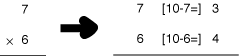illustration 3

Here there is a carry: the 1 in the 12 goes over to make 3 into 4.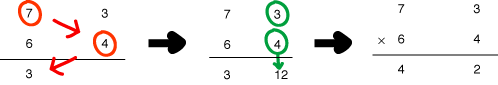illustration 4

B. Here's how to use VERTICALLY AND CROSSWISE for multiplying numbers close to 100. Suppose you want to multiply 88 by 98. Not easy, you might think. But with VERTICALLY AND CROSSWISE you can give the answer immediately, using the same method as above.

(a) Both 88 and 98 are close to 100. 88 is 12 below 100, and 98 is 2 below 100. You can imagine the sum set out like this: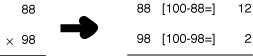illustration 5

(b) As before, the 86 comes from subtracting crosswise: 88 - 2 = 86 (or 98 - 12 = 86--you can subtract either way; you will always get the same answer). (c) And the 24 in the answer is just 12 x 2: you multiply vertically.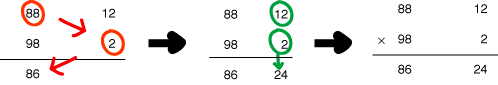illustration 6

So 88 x 98 = 8624.

This is so easy. It is just mental arithmetic.

Multiplying numbers just over 100. Look at 103 x 104 = 10712.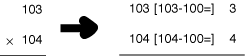illustration 7

107 is just 103 + 4 (or 104 + 3), and 12 is just 3 x 4.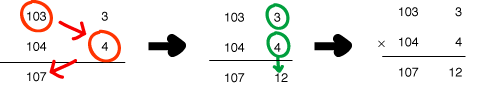illustration 8

The answer is in two parts: 107 and 12:  10712.

Similarly for calculating 107 x 106 = 11342. First find the difference between 107 and 100, and between 106 and 100: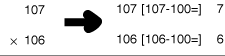illustration 9

107 + 6 = 113, and 7 x 6 = 42, as illustrated below: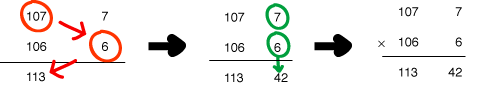illustration 10

C. The easy way to add and subtract fractions. Use VERTICALLY AND CROSSWISE to write the answer straight down!

(a) Multiply crosswise, and add to get the top of the answer: 2 x 5 = 10 and 1 x 3 = 3. (b) Then 10 + 3 = 13. (c) The bottom of the fraction is just 3 x 5 = 15. You multiply the bottom number together.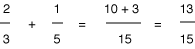illustration 11

Subtracting is just as easy: (a) multiply crosswise as before, (b) but then subtract: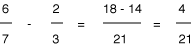illustration 12

#### 2. Using the formula BY ONE MORE THAN THE ONE BEFORE:

A. A quick way to square numbers that end in 5, using the formula BY ONE MORE THAN THE ONE BEFORE.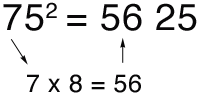illustration 13

75^2 means 75 x 75. The answer is in two parts: 56 and 25. (a) The last part is always 25. (b) The first part is the first number, 7, multiplied by the number "ONE MORE", which is 8.  7 x 8 = 56, and so the answer to 75^2 is 5625.

Similarly 85^2 = 7225 because 8 x 9 = 72.

B. Method for multiplying numbers where the first figures are the same and the last figures add up to 10, using the formula BY ONE MORE THAN THE ONE BEFORE.

Look at 32 x 38 = 1216. Both numbers 32 and 38 here start with 3, and the last
figures (2 and 8) add up to 10.

(a) So we just multiply 3 by 4 (the next number up) to get 12 for the first part of the answer. And (b) we multiply the last figures: 2 x 8 = 16 to get the last part of the answer. It works out like this: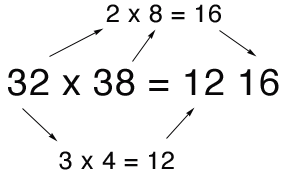illustration 14

And 81 x 89 = 7209. The same method applies.

As you can see, Vedic Math is not only incredibly efficient, practical and fast, it's really FUN!  This is so simply because the Vedic system taps into patterns of brain activity that are inherently natural.  Again, this is how the brain "wants to think".

In conclusion, I hope this little presentation into the wonderful world of Vedic Math will inspire the reader to investigate further.  As an educator, it is quite possibly one of the most satisfying and awe-inspiring scholastic journeys I have ever taken.  Anyone who pursues it thus will surely derive much satisfaction.

Of course, Vedic Math is only one aspect of the entirety of Vedic Culture per se, which was at one time the original, worldwide culture of the human race for countless millenniums.  It was not until His Divine Grace A.C. Bhaktivedanta Swami Prabhupada (the Founder Acharya of the International Society for Krishna Consciousness) first came to America in 1965 that the lost, ancient Vedic Culture was brought to the forefront of human awareness once again.  Srila Prabhupada single-handedly set into motion a renaissance of Vedic Culture.  Everything from its ancient philosophy, its spiritual food, its chanting and dancing to the Holy Names of Lord Krishna, to its art, architecture and music, Srila Prabhupada successfully transplanted the wonderfully practical way of "high thinking and plain living" all around the globe.  It is to him that this humble submission is offered.

Overview of Vedic Mathematics/ WORLD SANKIRTAN PARTY
Home | About | Events | World Sankirtan Party | Inside Nam Hatta
eBooks | Site Map | Store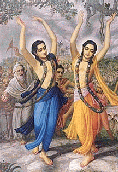Sri Guru and Gauranga

Related Articles

Related Topics

Education
Vedas - Vedic Authority